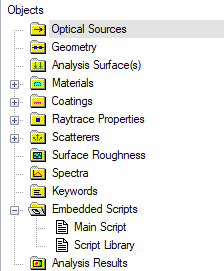# Embedded Script Libraries

In the tree view below, there are two embedded scripts.  It is the purpose of this article to demonstrate how subroutines and functions defined in the "Script Library" can be called from within the "Main Script".  In FRED, we will end up compiling the "Script Library" node into an Object that can then be referenced from within the "Main Script".In this example, the Script Library contains only one custom Function called "Add2".  Note that the Main subroutine is also included, but not used in this example (it doesn't hurt to have it there).  The Add2 function accepts two arguments, "in_v1" and "in_v2" which are Double precision floats.  The Add2 function returns one value "As Double".  The return value is simply the sum of the two input arguments.

```Sub Main

End Sub

Function Add2( ByVal in_v1 As Double, _
ByVal in_v2 As Double ) As Double

Return in_v1 + in_v2

End Function```

The goal is to call the Add2 function from within the Main Script.  In order to do this, three steps will be taken:

1. Use FindEmbeddedScript to get a node reference to the embedded script that will be compiled into a library
2. Use GetEmbeddedScriptLib to form a library Object
3. Use the "dot" notation on the Object to call its subroutines and functions

These three steps are taken in the Main subroutine of the "Main Script" as shown below.  Note how the Add2 function is called from the Object using "eLib.Add2()".

```Sub Main

'Compile embedded script into a library object
Dim eScript As Long, eLib As Object
eScript  = FindEmbeddedScript( "Script Library" )
Set eLib = GetEmbeddedScriptLib( eScript )

'Call a function out of the library
Dim sumVal As Double
sumVal = eLib.Add2( 5.21, 8.44 )

Print "Sum of values = " & sumVal

End Sub```

Example FRED File: Embedded Script Libraries